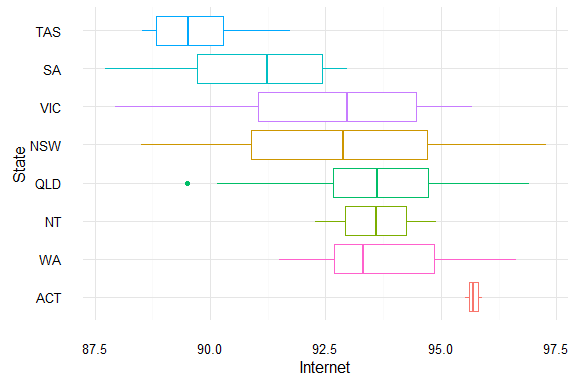# Introduction

eechidna (Exploring Election and Census Highly Informative Data Nationally for Australia) is an R package that makes it easy to look at the data from the 2011 Australian Census, and the 2013 Federal Election.

This vignette documents how to access the data from the 2011 Census. We show a few typical methods to explore the data.

## 2011 Census data

The data is loaded as abs2011 when you load eechidna. Let’s look take a brief glimpse of the data.

library(eechidna)
library(plyr)
library(dplyr)

glimpse(abs2011)
## Observations: 150
## Variables: 35
## $ID (fctr) 101, 102, 103, 104, 105, 106, 107, 108, 109... ##$ Electorate        (chr) "Banks", "Barton", "Bennelong", "Berowra", "...
## $State (chr) "NSW", "NSW", "NSW", "NSW", "NSW", "NSW", "N... ##$ Population        (int) 147760, 142069, 148707, 131371, 158408, 1430...
## $Area (dbl) 44.6388, 40.3341, 58.6107, 745.6880, 60.7705... ##$ MedianIncome      (int) 537, 558, 624, 702, 385, 775, 522, 522, 491,...
## $Unemployed (dbl) 6.1, 5.7, 5.7, 4.2, 9.4, 4.8, 5.1, 5.2, 8.6,... ##$ Bachelor          (dbl) 13.360179, 12.721987, 18.823593, 17.275502, ...
## $Postgraduate (dbl) 4.6974824, 4.1662854, 7.6654092, 5.7212018, ... ##$ Christianity      (dbl) 59.89172, 62.45416, 59.32202, 66.92649, 49.3...
## $Catholic (dbl) 24.70154, 25.16946, 27.35513, 26.12296, 26.6... ##$ Buddhism          (dbl) 5.4270439, 3.9649748, 4.5814925, 2.6543149, ...
## $Islam (dbl) 4.9756362, 8.5183960, 2.1007754, 1.2818659, ... ##$ Judaism           (dbl) 0.15430428, 0.21538830, 0.32210992, 0.289257...
## $NoReligion (dbl) 19.782756, 13.741210, 22.861062, 19.978534, ... ##$ Age00_04          (dbl) 6.154575, 6.521479, 5.928436, 5.834621, 8.18...
## $Age05_14 (dbl) 11.767055, 11.075604, 11.033105, 14.055613, ... ##$ Age15_19          (dbl) 6.197212, 5.305169, 5.965422, 7.746002, 7.02...
## $Age20_24 (dbl) 7.160260, 6.465168, 8.349977, 6.384210, 7.31... ##$ Age25_34          (dbl) 14.648078, 16.128079, 15.028882, 8.705118, 1...
## $Age35_44 (dbl) 13.71819, 15.16024, 14.40215, 13.89728, 13.1... ##$ Age45_54          (dbl) 14.18517, 12.95497, 14.03498, 16.33009, 12.7...
## $Age55_64 (dbl) 10.975230, 10.711696, 10.903320, 13.368247, ... ##$ Age65_74          (dbl) 7.293584, 7.639950, 6.850384, 8.006333, 6.09...
## $Age75_84 (dbl) 5.362750, 5.532523, 5.177967, 3.972718, 4.42... ##$ Age85plus         (dbl) 2.5378993, 2.5051208, 2.3240332, 1.6997663, ...
## $BornOverseas (dbl) 5.5312669, 9.3912113, 5.9344886, 4.5040382, ... ##$ Indigenous        (dbl) 0.6774499, 0.5476212, 0.3570780, 0.3760343, ...
## $EnglishOnly (dbl) 7.095290, 7.603348, 9.403727, 13.687952, 4.8... ##$ OtherLanguageHome (dbl) 47.860043, 54.082875, 42.427727, 21.679062, ...
## $Married (dbl) 39.76245, 39.38650, 40.09562, 45.28625, 35.7... ##$ DeFacto           (dbl) 3.768273, 4.418980, 4.036125, 3.300576, 2.37...
## $FamilyRatio (dbl) 51.43814, 50.69508, 51.31702, 50.65730, 50.2... ##$ Internet          (dbl) 93.30198, 92.71973, 94.80320, 96.93996, 92.2...
## \$ NotOwned          (dbl) 76.37797, 76.29182, 76.53475, 72.43367, 81.4...

Here we see that we have 150 observations and 35 variables.

Each observation is data pertaining to a particular federal electorate as described by http://www.aec.gov.au/profiles/.

Each column is now described here:

Variable Details
ID Commonwealth Electoral District identifier
Electorate Name of electorate
State State containing electorate
Population Total population of electorate
Area Area of electorate in square kilometres
MedianIncome Median income of people within electorate
Unemployed Percentage of people unemployed
Bachelor Percentage of people whose highest qualification is a Bachelor degree
Christianity Percentage of people affiliated with the Christian religion (of all denominations)
Catholic Percentage of people affiliated with the Catholic denomimation.
Buddhism Percentage of people affiliated with the Buddhist religion.
Islam Percentage of people affiliated with the Islam religion.
Judaism Percentage of people affiliated with the Jewish religion.
NoReligion Percentage of people with no religion.
Age00_04 Percentage of people aged 0-4.
Age05_14 Percentage of people aged 5-9.
Age15_19 Percentage of people aged 15-19.
Age20_24 Percentage of people aged 20-24.
Age25_34 Percentage of people aged 25-34.
Age35_44 Percentage of people aged 35-44.
Age45_54 Percentage of people aged 45-54.
Age55_64 Percentage of people aged 55-64.
Age65_74 Percentage of people aged 65-74.
Age75_84 Percentage of people aged 75-84.
Age85plus Percentage of people aged 85 or higher.
BornOverseas Percentage of people born outside Australia.
Indigenous Percentage of people who are Indigenous
EnglishOnly Percentage of people who speak only English
OtherLanguageHome Percentage of people who speak a language other than English at home
Married Percentage of people who are married
DeFacto Percentage of people who are in a de facto marriage
FamilyRatio Total number of families to total number of people (times 100)
Internet Percentage of people with home internet
NotOwned Percentage of dwellings not owned (either outright or with a mortgage)

So let’s just look at some nice and simple plots using ggplot2.

## Unemployment

library(ggplot2)

ggplot(data = abs2011,
aes(x = Unemployed)) +
geom_density(fill = "salmon",
bw = "SJ",
colour = NA) +
geom_rug(colour = "salmon") +
theme_minimal() +
xlim(0, 12)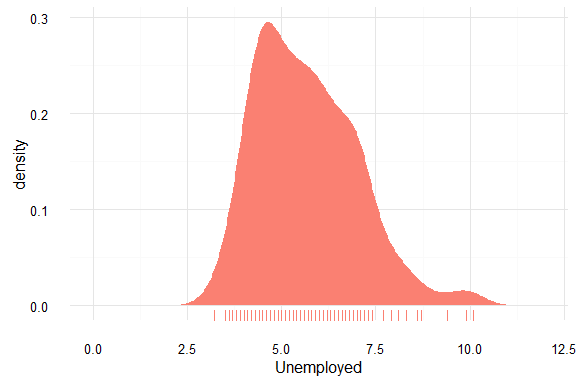## Unemployment by state

ggplot(data = abs2011,
aes(x = reorder(State, -Unemployed),
y = Unemployed,
colour = State)) +
geom_boxplot() +
labs(x = "State",
y = "% Unemployment") +
theme_minimal() +
theme(legend.position = "none")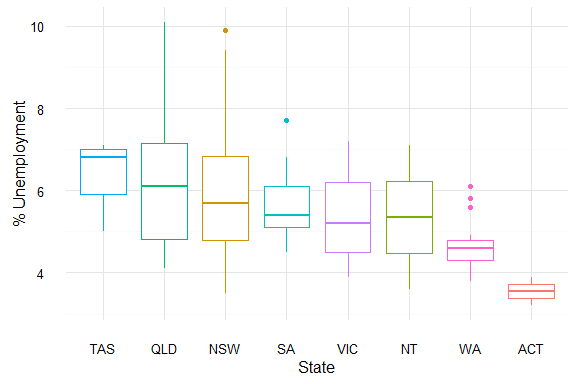## Age

ggplot(data = abs2011,
aes(x = Age00_04)) +
geom_density(fill = "steelblue",
bw = "SJ",
colour = NA) +
xlim(3,11) +
geom_rug(colour = "steelblue") +
theme_minimal() +
labs(x = "% Aged between 0 and 4")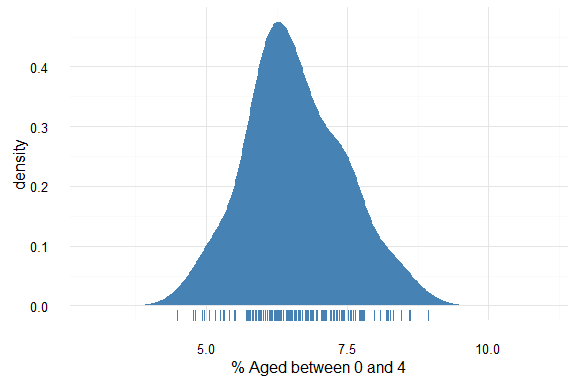ggplot(data = abs2011,
aes(x = reorder(State, -Age00_04),
y = Age00_04,
colour = State)) +
geom_boxplot() +
theme_minimal() +
labs(x = "State",
y = "% Aged between 0 and 4") +
theme(legend.position = "none") +
coord_flip()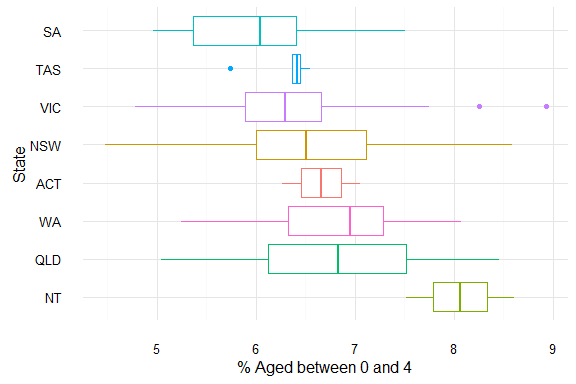However, there are many age groups. To look at all of them at once, we can gather them into a dataframe ready for plotting using tidyr.

library(tidyr)

abs2011 %>%
select(starts_with("Age"),
Electorate) %>%
gather(key = "Age",
value = "Percent_in_electorate",
-Electorate) %>%
ggplot(data = .,
aes(x = reorder(Age, - Percent_in_electorate),
y = Percent_in_electorate,
colour = Age)) +
geom_boxplot() +
coord_flip() +
theme_minimal() +
theme(legend.position = "none") +
labs(x = "Age Groups",
y = "% in Electorate")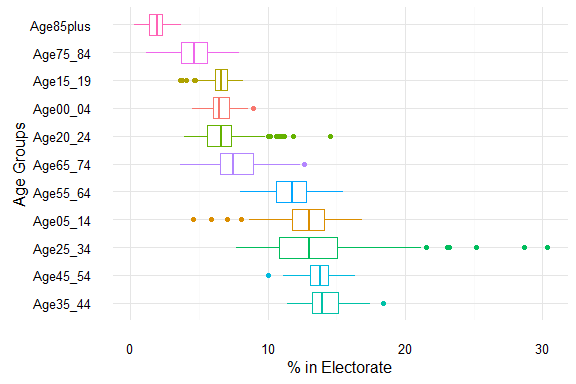## Income

ggplot(data = abs2011,
aes(x = MedianIncome)) +
geom_density(fill = "salmon",
bw = "SJ",
colour = NA) +
xlim(250,1100) +
geom_rug(colour = "salmon") +
theme_minimal()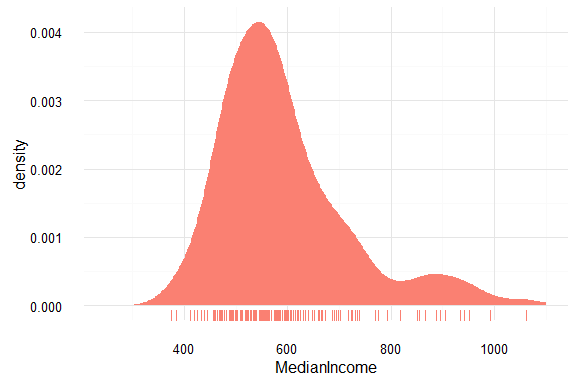## Income by State

ggplot(data = abs2011,
aes(x = reorder(State, -MedianIncome),
y = MedianIncome,
colour = State)) +
geom_boxplot() +
theme_minimal() +
theme(legend.position = "none") +
labs(x = "State")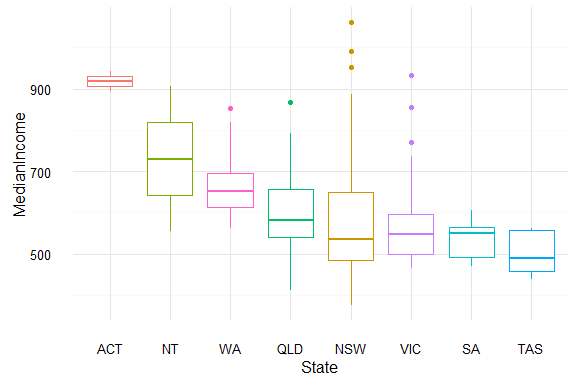If you’re intersted in getting a sense of the distribution of the data, you can add in the points to get a bit more of a sense on the distribution.

ggplot(data = abs2011,
aes(x = reorder(State, -MedianIncome),
y = MedianIncome,
colour = State)) +
geom_boxplot() +
geom_jitter(alpha = 0.35,
size = 2,
width = 0.3) +
theme_minimal() +
theme(legend.position = "none") +
labs(x = "State")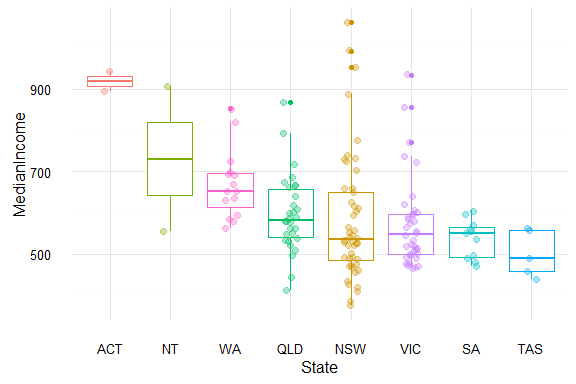## Education

##### Bachelor
ggplot(data = abs2011,
aes(x = Bachelor)) +
geom_density(fill = "salmon",
bw = "SJ",
colour = NA) +
geom_rug(colour = "salmon") +
theme_minimal() +
labs(x = "% of electorate with a Bachelor degree") +
xlim(0, 30)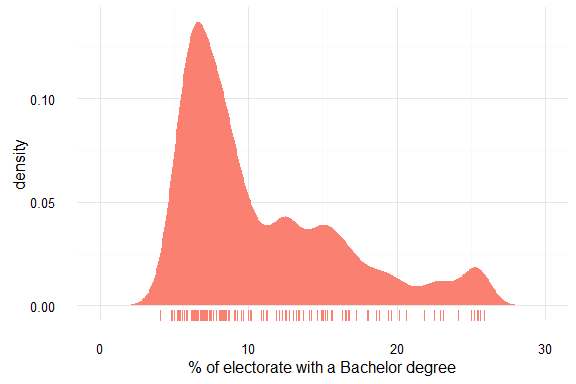##### Bachelor by state
ggplot(data = abs2011,
aes(x = reorder(State, -Bachelor),
y = Bachelor,
colour = State)) +
geom_boxplot() +
theme_minimal() +
labs(x = "State") +
theme(legend.position = "none")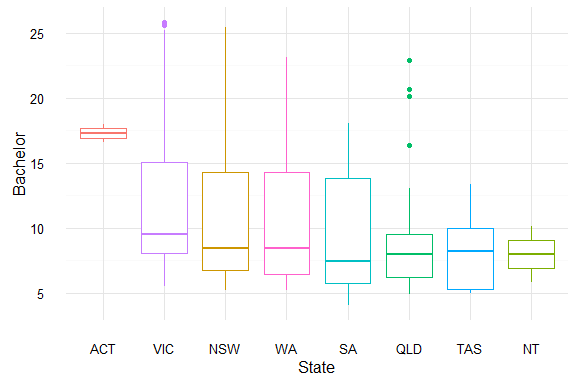##### Bachelor and income
ggplot(data = abs2011,
aes(x = Bachelor,
y = MedianIncome)) +
geom_point(colour = "steelblue",
alpha = 0.75) +
theme_minimal()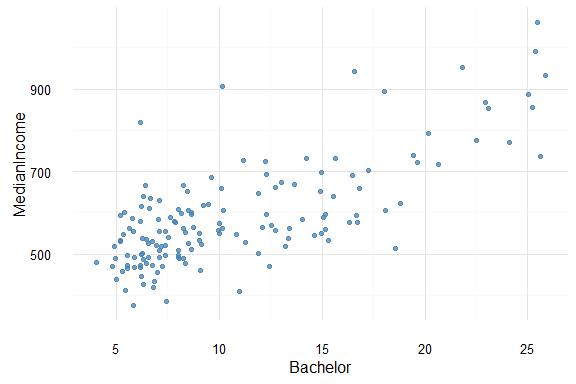ggplot(data = abs2011,
colour = State)) +
geom_boxplot() +
theme_minimal() +
labs(x = "State") +
theme(legend.position = "none")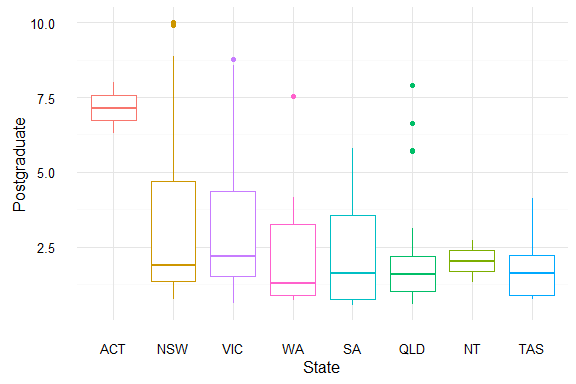ggplot(data = abs2011,
y = MedianIncome)) +
geom_point(colour = "steelblue",
alpha = 0.75) +
theme_minimal()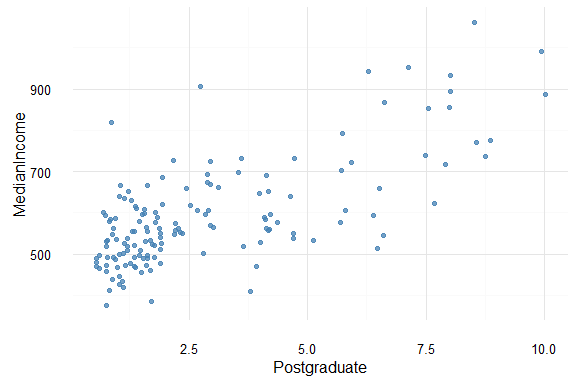##### Comparing income across Bachelors and postgraduate
abs2011 %>%
Bachelor,
MedianIncome) %>%
gather(key = "Education",
value = "Prop_Educated",
-MedianIncome) %>%
ggplot(data = ,
aes(x = Prop_Educated,
y = MedianIncome,
colour = Education)) +
geom_point() +
geom_smooth() +
theme_minimal() +
scale_color_brewer(type = "qual", palette = "Set1")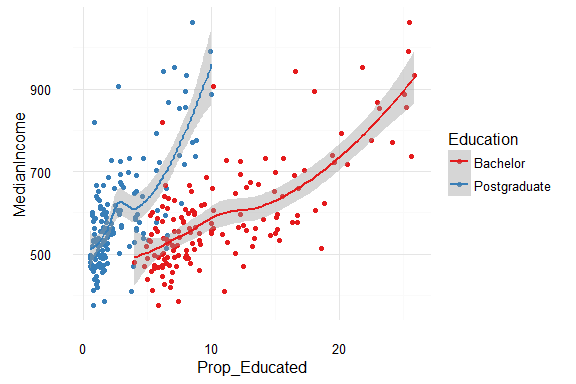# theme(legend.position = "bottom",
#       legend.direction = "vertical")

## Religion

Let’s look at all of the religions

abs2011 %>%
select(Christianity,
Catholic,
Buddhism,
Islam,
Judaism,
NoReligion) %>%
gather(key = "ReligionType",
value = "Percent") %>%
ggplot(data = .,
aes(x = reorder(ReligionType, -Percent),
y = Percent,
colour = ReligionType)) +
geom_boxplot() +
theme_minimal() +
theme(legend.position = "none") +
coord_flip() +
labs(x = "Religion")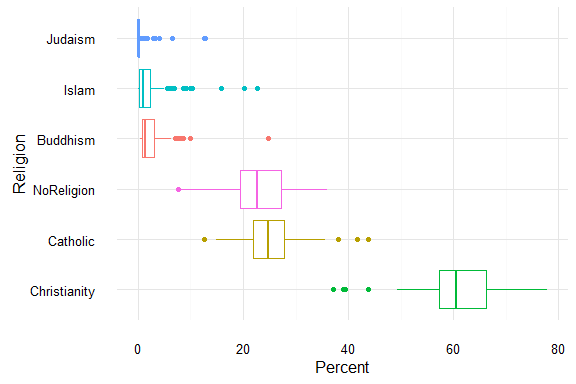##### Christianity by State
ggplot(data = abs2011,
aes(x = reorder(State, -Christianity),
y = Christianity,
colour = State)) +
geom_boxplot() +
theme_minimal() +
theme(legend.position = "none") +
coord_flip() +
labs(x = "State")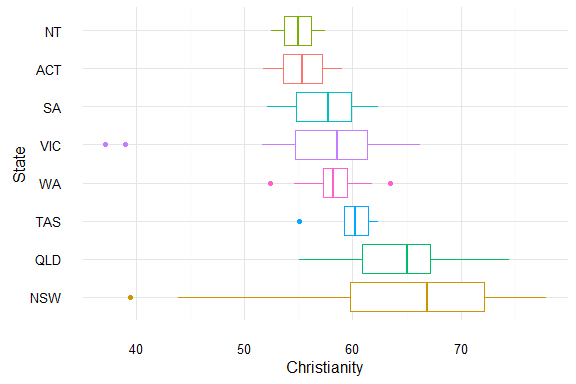## Internet

ggplot(data = abs2011,
aes(x = Internet)) +
geom_density(fill = "steelblue",
bw = "SJ",
colour = NA) +
geom_rug(colour = "steelblue") +
theme_minimal() +
labs(x = "% of electorate with Internet") +
xlim(85, 100)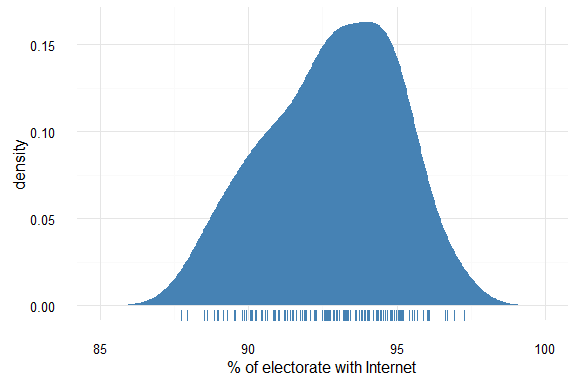## Internet by state

ggplot(data = abs2011,
aes(x = reorder(State, -Internet),
y = Internet,
colour = State)) +
geom_boxplot() +
theme_minimal() +
theme(legend.position = "none") +
coord_flip() +
labs(x = "State")